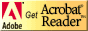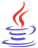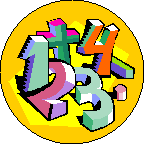Standardized Test Preparation and Tips for Success

Strand Resources: Number, Number Sense, and OperationsCT4ME has identified resources related to each benchmark of the Ohio Mathematics Academic Content Standards that students should have mastered by the end of the grades 8-10 program.

Identify the benchmark (A-I) for review in Number, Number Sense, and Operations.  Below the benchmark, you will find Web resources for reviewing the concept and practice problems.

Students should be able to review on their own using these resources.  If you are not an Ohio educator, these resources can still benefit your test prep efforts, as benchmarks in other states are similarly stated.

Number, Number Sense and Operations (pdf)  This test prep booklet (about 200 KB) is designed to help you monitor your progress toward mastery of each benchmark in this strand.  You can print the entire booklet, or just those pages for benchmarks you need to work on.  Write notes in the booklet next to each resource you use in your review.If needed, download Adobe Acrobat Reader, free software for viewing and printing PDF files.You might need to download Java, a free plug-in to interact with virtual manipulatives on this page.Other strand resources:

Number, Number Sense, and Operations ]   [ Measurement ]   [ Geometry and Spatial Sense ]   [ Patterns, Functions, and Algebra ]   [ Data Analysis and Probability ]   [ Mathematical Processes ]How shall we interpret Number?Here's a bit of history for you!

Imagine writing your numbers with seven different symbols: a single stroke, a hobble for cattle, a coil of rope, a lotus plant, a finger, a tadpole or frog, or a god with his arms raised above his head.  Read more on this topic from Mark Millmore and explore doing a few problems with Egyptian Math.

Benchmarks for Number, Number Sense, and Operations

Benchmarks in this strand have been correlated to the Common Core State Standards (CCSS) for Mathematics for the Conceptual Category (CC), Domain (D), and Cluster (C).NOTE: As a general resource for Number Sense and Operations strand benchmarks, teachers might consult the following resource:

• Learning Math: Number and Operations from Annenberg Learner contains 10 sessions each about 2.5 hours long with videos, online and print-based problem solving activities, interactive activities and demonstrations.

A.  Use scientific notation to express large numbers and numbers less than one.

CCSS: CC: grade 8, D: Expressions and Equations, C: Work with radicals and integer exponents.

AlgebraLab.org: Scientific Notation Introduction and practice exercises converting to scientific notation and decimal notation from the scientific form.

West Texas A&M University Virtual Math Lab College Algebra Tutorial: Scientific Notation: How to write numbers in scientific notation, perform multiplication and division with video explanations and practice problems.

Math and Science Activity Center from Edinformatics.com:  Scientific Notation Problem Generator.  Randomly generated problems in either scientific notation or standard notation representation of a number will be shown.  Fill in the blanks, then check answer.

QuickMath.com: Scientific Notation.  Enter your number and see detailed explanation of a conversion.Play the YouTube video from the Ohio Resource Center Tutorials for High School Mathematics:

B.  Identify subsets of the real number system.

Learner.org Learning Math: Number Sets, Infinity and Zero.  Explore number sets that make up the real number system and look more closely at the concept of infinity and the importance of zero.

Lumen Prealgebra: Identifying Rational and Irrational NumbersPlay video at SchoolTube.com for Real Numbers and Their Graphs.

C.  Apply properties of operations and the real number system, and justify when they hold for a set of numbers.

CCSS: CC: Algebra, D: Arithmetic with Polynomials and Rational Expressions, C: Perform arithmetic operations on polynomials.

Maths Online Interactive Test: Number Sets (multiple choice with more than one answer).

Purple Math: Order of Operations (PEMDAS) Lesson with worked examples.

Math.com: Order of Operations with more than one operation. Lesson, worked examples, and practice problems.

West Texas A&M University, Intermediate Algebra Tutorials: Operations on Real Numbers, practice with answers, and additional related linksPlay videos at YouTube.com for Real Numbers:

D.  Connect physical, verbal and symbolic representations of integers, rational numbers and irrational numbers.

CCSS: CC: grade 8, D: Number System, C: Know that there are numbers that are not rational, and approximate them by rational numbers.

The Math Page, Real Numbers:

E.  Compare, order and determine equivalent forms of real numbers.

CCSS: CC: grade 7, D: Number System, C: Apply and extend previous understandings of operations with fractions to add, subtract, multiply, and divide rational numbers.

CCSS: CC: grade 7, D: Expressions and Equations, C: Solve real-life and mathematical problems using numerical and algebraic expressions and equations.

CCSS: CC: grade 8, D: Number System, C: Know that there are numbers that are not rational, and approximate them by rational numbers.

MathisFun.com: Explanation for converting fractions, decimals, and percents with practice

AAA Math: Comparing and equivalent forms (rational numbers) with online practice

F.  Explain the effects of operations on the magnitude of quantities.

NCTM Illuminations: Select E-examples 6.3.1 and 6.3.2: Learning about Length, Perimeter, Area, and Volume of Similar Objects by Using Interactive Figures has two parts (JAVA required):

• 6.3.1 Side Length and Area of Similar Figures

• 6.3.2 Side Length, Volume, and Surface Area of Similar Solids

G.  Estimate, compute and solve problems involving real numbers, including ratio, proportion and percent, and explain solutions.

CCSS: CC: Geometry, D: Circles, C: Find arc lengths and areas of sectors of circles.

Algebra: Proportion Basics from Wyzant.com

AlgebraLab.org:

The Math Page, Skill in Arithmetic: Percent increase or decrease problems with answers

Shodor.org: Percents

H.  Find the square root of perfect squares, and approximate the square root of non-perfect squares.

CCSS: CC: grade 8, D: Expressions and Equations, C: Work with radicals and integer exponents.

I.  Estimate, compute and solve problems involving scientific notation, square roots and numbers with integer exponents.

CCSS: CC: grade 8, D: Expressions and Equations, C: Work with radicals and integer exponents.

Institute for Energy and Environmental Research: Scientific Notation Review and Practice Worksheet with answers

Play the YouTube video from the Ohio Resource Center Tutorials for High School Mathematics:

• Scientific Notation: Computation for computing with scientific notation, using significant digits, and explaining the meaning of precision and accuracy.

• Exponents for understanding exponents and using the rules for computation.Are you ready for the test?

References

*Ohio Mathematics Academic Content Standards (2001). http://www.ode.state.oh.us/GD/Templates/Pages/ODE/ODEPrimary.aspx?page=2&TopicID=1696&TopicRelationID=1704 [URL is no longer active.]

Ohio Mathematics Academic Content Standards Crosswalk, K-8 and High School (2010).  https://web.archive.org/web/20180206120934/http://education.ohio.gov/getattachment/Topics/Ohio-s-New-Learning-Standards/Mathematics/Transition-Tools-Ohio-s-New-Learning-Standards-K-1/Alignment-Toolkit-Phase-1-Gap-Analysis/HS-crosswalk-mathematics-4-17-12.pdf.aspx [Revised 4/17/2012].

*Note 2019: The current academic content standards for mathematics are now available at http://education.ohio.gov/Topics/Learning-in-Ohio/Mathematics/Ohio-s-Learning-Standards-in-MathematicsSee other strand resources:See related pages: [ Standardized Test Preparation and Tips for Success (first  page)]  [ OGT Introduction ]